# SSAT Upper Level Math : How to find slope of a line

## Example Questions

← Previous 1 3

### Example Question #1 : How To Find Slope Of A Line

One end of a board that is four feet long is on the ground. The other end is balanced on a box that is one foot tall, creating a slope. What is the slope of the board?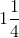Explanation:

The slope of a line is equal to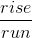Given that the box is one foot tall, the rise will be equal to "1."

Given that the board is four feet long, the run will be equal to "4."

Therefore, the slope is equal to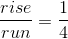.

### Example Question #23 : Other Lines

A line is given with the equation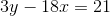. What is the slope of this line?Explanation:

To find the slope, put the equation inform.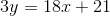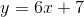Since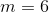, that must be the slope of the line.

### Example Question #24 : Other Lines

A line has the equation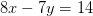. What is the slope of the line?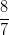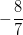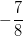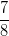Explanation:

Change the equation into the more familiar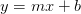form. The value ofwill be the slope.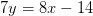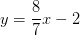### Example Question #2 : How To Find Slope Of A Line

What is the slope of a line that passes through the points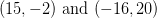?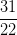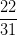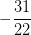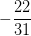Explanation:

Use the following formula to find the slope: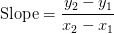Plug in the given points to find the slope.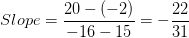### Example Question #3 : How To Find Slope Of A Line

Find the slope of the line that passes through the points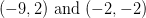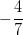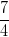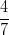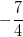Explanation:

Use the following formula to find the slope: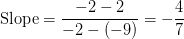### Example Question #4 : How To Find Slope Of A Line

Find the slope of the line that passes through the points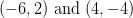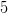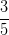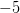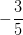Explanation:

Use the following formula to find the slope: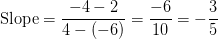### Example Question #21 : Lines

Find the slope of the line that passes through the points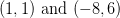.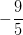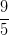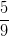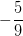Explanation:

Use the following formula to find the slope: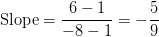### Example Question #5 : How To Find Slope Of A Line

Find the slope of the line that passes through the points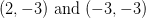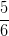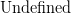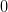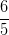Explanation:

Use the following formula to find the slope: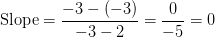### Example Question #6 : How To Find Slope Of A Line

Find the slope of the line that passes through the points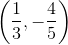and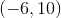.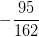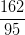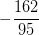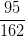Explanation:

Use the following formula to find the slope:Plug in the given points to find the slope.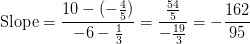### Example Question #81 : Geometry

A line goes passes through the points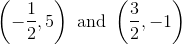. What is the slope of this line?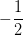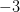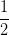Explanation:

Use the following formula to find the slope of a line:The slope of this line would be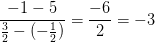← Previous 1 3